Courses

AC Voltage Controllers & Cyclo Converters Notes | Study Power Electronics - Electrical Engineering (EE)

Electrical Engineering (EE): AC Voltage Controllers & Cyclo Converters Notes | Study Power Electronics - Electrical Engineering (EE)

The document AC Voltage Controllers & Cyclo Converters Notes | Study Power Electronics - Electrical Engineering (EE) is a part of the Electrical Engineering (EE) Course Power Electronics.
All you need of Electrical Engineering (EE) at this link: Electrical Engineering (EE)

Cycloconverters

In Figs. 3.2 and 3.3, the two typical types of cycloconverters are presented. In the first case there are two three-phase midpoint controlled rectifiers connected back to back. The second case shows two three-phase bridge rectifier converters connected back to back. Both are used for three-phase to three-phase conversion. In Fig. 3.4 the single-phase output voltage and current waves are presented for the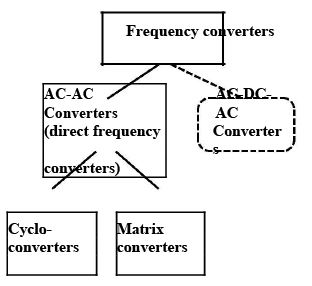FIGURE 3.1 Classification of frequency converters.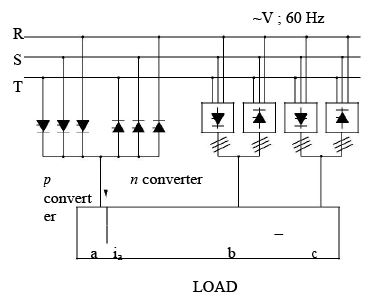FIGURE 3.2 Cycloconverter scheme with three-phase midpoint controlled rectifier.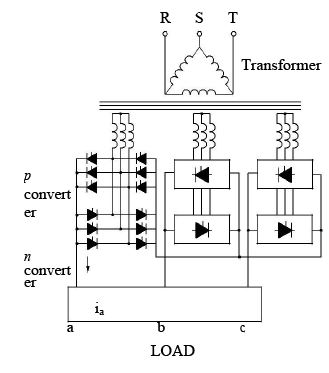FIGURE 3.3 Cycloconverter scheme with three-phase bridge controlled rectifier.

bridge rectifier circuits. The output voltage Vand current ia have Va1 and ia1 fundamental components with φ1 phase displacement and numerous harmonics. Because of the load inductance, the current harmonics will be significantly lower than the voltage harmonics. The firing angles are αP and αN for the p and n converters, respectively. In general, the controls are designed so that only the thyristors of either the p or n converter is firing, which produces a current in the desired direction. During this period the other converter is blocked. When the current changes direction, both converters must be blocked for a short time.
It is possible to operate without blocking the converters. In this case, their average voltage must be the same, and therefore the relation αp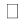180− αn is valid. However, additional inductances are necessary to limit the circulating currents between two converters since the instantaneous voltages of the two converters differ from one another.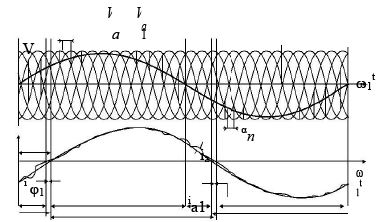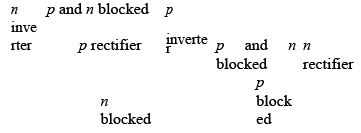FIGURE 3.4 Voltage and current vs. time for cycloconverter with three-phase bridge converters.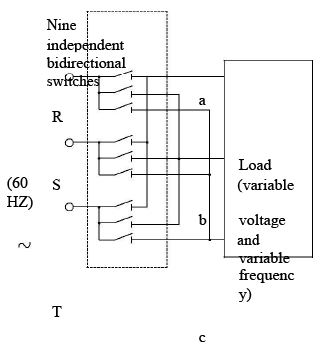FIGURE 3.5 Three-phase to three-phase matrix converter.

The phase control of the p and n converters is modulated by a sine or trapezoidal wave. The content of the harmonics for sine modulation is lower; however, the maximum value of the output voltage is lower than that for trapezoidal modulation. During every cycle of the output voltage both of the converters must work as rectifiers and inverters.
The shape of the output voltage goes from bad to worse with an increase in the output voltage and the output frequency. If the frequency reaches the well-defined value the current harmonics become unacceptable. This frequency is usually 33% of supply frequency for three-phase midpoint ( Fig. 3.2) and 50% for three-phase bridge ( Fig. 3.3) converters.
The cycloconverter is usually used for three-phase, high-power, low-speed synchronous motor drives and rarely employed for induction motor drives.

AC VOLTAGE CONTROLLERS

Introduction

AC to AC voltage converters operates on the AC mains essentially to regulate the output voltage. Portions of the supply sinusoid appear at the load while the semiconductor switches block the remaining portions. Several topologies have emerged along with voltage regulation methods, most of which are linked to the development of the semiconductor devices.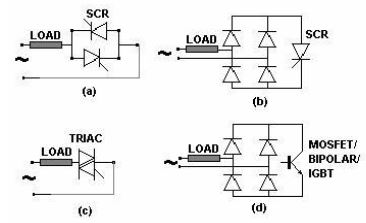Fig 26.1 Some single phase AC-AC voltage regulator topologies. (a) Back-to -back SCR; (b) One SCR in (a) replaced by a four-diode full wave diode bridge; (c) A bi-directionally conducting TRIAC; (d) The SCR in (b) replaced by a transistor.

The regulators in Fig 26.1 (a), (b) and (c) perform quite similarly. They are called Phase Angle Controlled (PAC) AC-AC converters or AC-AC choppers. The TRIAC based converter may be considered as the basic topology. Being bi-directionally conducting devices, they act on both polarities of the applied voltage. However, dvdtre −applied their ratings being poor, they tend to turn-on in the opposite direction just subsequent to their turn-off with an inductive load. The 'Alternistor' was developed with improved features but was not popular. The TRIAC is common only at the low power ranges. The (a) and (b) options are improvements on (c) mostly regarding current handling and turn-off-able current rating.

A transistorised AC-AC regulator is a PWM regulator similar to the DC-DC converters. It also requires a freewheeling path across the inductive load, which has also got to be bi-directional. Consequently, only controlled freewheeling devices can be used.

Fig. 26.2 illustrates the operation of the PAC converter with a resistive load. The device(s) is triggered at a phase-angle 'α' in each cycle. The current follows the voltage wave shape in each half and extinguishes itself at the zero crossings of the supply voltage. In the two-SCR topology, one SCR is positively biased in each half of the supply voltage. There is no scope for conduction overlap of the devices. A single pulse is sufficient to trigger the controlled devices with a resistive load. In the diode-SCR topology, two diodes are forward biased in each half. The SCR always receives a DC voltage and does not distinguish the polarity of the supply. It is thus always forward biased. The bi-directional TRIAC is also forward biased for both polarities of the supply voltage.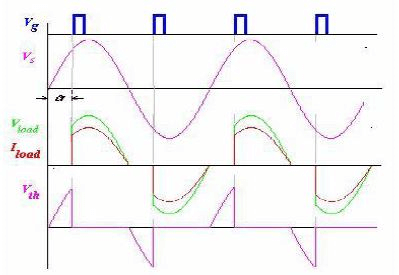Fig. 26.2 Operation of a Phase Angle Controlled AC-AC converter with a resistive load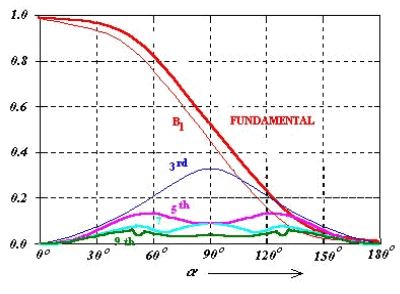Fig. 26.3 The rms output voltage and the most important harmonics versus triggering angle α.

As is evident from the current waveforms, the PAC introduces significant harmonics both into the load and the supply. This is one of the main reasons why such controllers are today not acceptable. The ideal waveform as shown in Fig 26.2 is half wave symmetric. However it is to be achieved by the trigger circuits. The controller in Fig. 26.4 ensures this for the TRIAC based circuit. While the TRIAC has a differing characteristic for the two polarities of biasing with the 32V DIAC - a two terminal device- triggering is effected when the capacitor voltage reaches 32 V. This ensures elimination of DC and even components in the output voltage.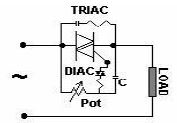Fig. 26.4 DIAC based trigger circuit for a TRIAC to ensure symmetrical triggering in the two halves of the supply.
For the SCR based controllers, identical comparators for the two halves of the AC supply, which generates pulses for the two SCRs ensures DC and even harmonic free operation.
The PAC operates with a resistive load for all values of α ranging from 0o
The fundamental current, if can be represented as

In machine drives it is only the fundamental component, which is useful. However, in resistance heating type of application all harmonics are of no consequence. The corrupted supply current nevertheless is undesirable.

Power Factor

The power factor of a nonlinear deserves a special discussion. Fig. 26.2 shows the supply voltage and the non-sinusoidal load current. The fundamental load/supply current lags the supply voltage by the φ1, 'Fundamental Power Factor' angle. Cosφ1 is also called the 'Displacement Factor'. However this does not account for the total reactive power drawn by the system. This power factor is inspite of the actual load being resistive! The reactive power is drawn also y the trigger-angle dependent harmonics. Now

The portion within square brackets in Eq. 26.5 is identical to the first part of the expression within brackets in Eq. 26.1, which is called the Fourier coefficient 'B1B'. The rms load voltage can also be similarly obtained by integrating between α and π and the result can be combined with Eq. 26.5 to give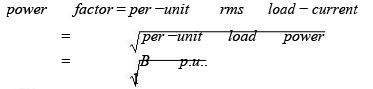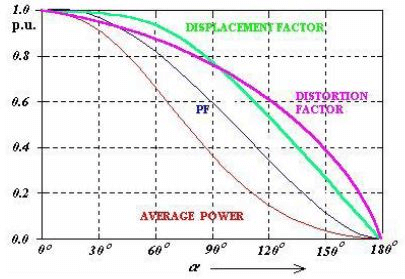Fig. 26.5 Variation of various performance parameters with triggering angle

With inductive loads the operation of the PAC is illustrated in Fig 26.5. The current builds up from zero in each cycle. It quenches not at the zero crossing of the applied voltage as with the resistive load but after that instant. The supply voltage thus continues to be impressed on the load till the load current returns to zero. A single-pulse trigger for the TRIAC 26.1 (c) or the anti-parallel SCR (b) has no effect on the devices if it (or the anti-parallel device) is already in conduction in the reverse direction. The devices would fail to conduct when they are intended to, as they do not have the supply voltage forward biasing them when the trigger pulse arrives. A single pulse trigger will work till the trigger angle α > φ, where φ is the power factor angle of the inductive load. A train of pulses is required here. The output voltage is controllable only between triggering angles φ and 180o.
The load current waveform is further explained in Fig. 26.6. The current is composed of two components. The first is the steady state component of the load current, iss and the second, itr is the transient component.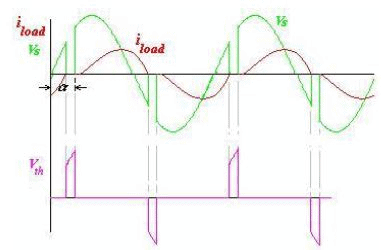Fig. 26.6 Operation of a single phase PAC with an inductive load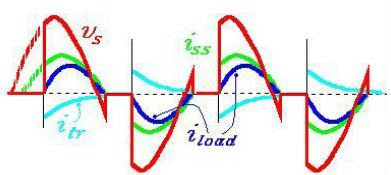Fig 26.7 Load current for a single phase AC-AC converter with a R_L load. Vs - supply voltage, iss -steady state current component , itr - transient current component and iload - load current (= iss + itr).

With an inductance in the load the distinguishing feature of the load current is that it must always start from zero. However, if the switch could have permanently kept the load connected to the supply the current would have become a sinusoidal one phase shifted from the voltage by the phase angle of the load, φ. This current restricted to the half periods of conduction is called the 'steady-state component' of load current iss. The 'transient component' of load current itr, again in each half cycle, must add up to zero with this iss to start from zero. This condition sets the initial value of the transient component to that of the steady state at the instant that the SCR/TRIAC is triggered. Fig. 26.6 illustrates these relations.

When a device is in conduction, the load current is governed by the equation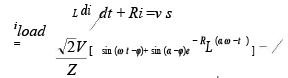Since at t = 0, iload = 0 and supply voltage v= √2Vsinωt the solution is of the form
The instant when the load current extinguishes is called the extinction angle β. It can be inferred that there would be no transients in the load current if the devices are triggered at the power factor angle of the load. The load current I that case is perfectly sinusoidal.

AC-AC Chopper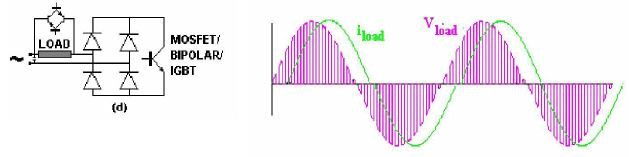Fig. 26.8 A complete Transitorised AC-AC chopper topology of the version shown in Fig. 26.1 and the corresponding load voltage and current waveforms for an inductive load. The output voltage is shown to be about 50% for a 0.5 Duty Ratio chopping.

The AC-AC converter shown in Fig 26.1 has to be augmented with two additional controlled devices clamping the load as indicated in Fig. 26.7. A large capacitor across the supply terminals is also to be inserted. These devices which are mostly transistors of the same variety as used for the chopper are necessary to clamp the voltages generated by the switching-off of the current carrying inductors in the load while the input capacitor takes care of the line inductances. The harmonics in the line current and load voltage waveforms are significantly different from those with the PACs. Mostly switching frequency harmonics are present in both the waveforms.

PAC as a static switch

Both single phase and three phase PACs are often used as static switches for applications like switching on of highly inductive loads without transients or for regulating output AC voltages by switching in tapings of a transformer. Such sequence control PACs while controlling the output voltage also permit improvement of the power factor as seen by the source. Sequence control can be two or multiple phase depending upon the application. Typical load voltage and current waveforms are shown in Fig. 26.8. The outer TRIACs connected to thwe higher voltage leads of the input transformer are triffered at the desired angle α, to realize the required load voltage. Obviously this voltage is greater than that available at the low voltage terminal of the transformer. This device continues conduction into the next half of the supply voltage till the load current falls to zero. The inner TRstarts conduction subsequently, requiring a wide pulse or a train of pulses. TR1 can be however triggered by a single pulse.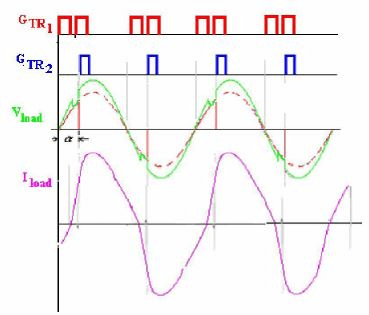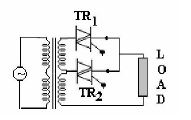Fig. 26.9 Load voltage and current control with a two-stage sequence control

Practice Questions and Problems with Answers

Q1.A single-phase transformer, assumed to have a negligible resistance compared to its inductance is switched on via a PAC. At what trigger angle will the operation be free fromtransients?
A1.
φ≈ 90ο
Therefore for transient free operation α = 90ο

Q2. For the load described in Q1, the PAC is triggered by a single pulse at α = 60ο . Sketch the load current waveform.

A2.  Since α < φL, the load current should have been continuous. However, the current in the SCR first triggered extinguishes at a β the total load current, i= iss + itr = 0. For this load which can be considered to be highly inductive β ≈ 360ο, say ≈ 360ο. Thus the first SCR conducts till that angle. The anti-parallel SCR is triggered at α = 60ο corresponding to a β ≈ 180 + 60 = 240ο when it is still reverse biased. It fails to conduct. The load thus sees only a unipolar current. The load current and voltage waveforms are illustrated in Fig 26. A2. Note that both the load voltage and current waveforms contain DC components.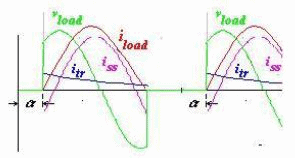Fig. 26. A2 The load current waveform and its steady-state and transient components when a highly inductive load is switched using single narrow trigger pulses.

The document AC Voltage Controllers & Cyclo Converters Notes | Study Power Electronics - Electrical Engineering (EE) is a part of the Electrical Engineering (EE) Course Power Electronics.
All you need of Electrical Engineering (EE) at this link: Electrical Engineering (EE)Use Code STAYHOME200 and get INR 200 additional OFF Use Coupon Code

Top Courses for Electrical Engineering (EE)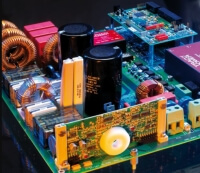Power Electronics

5 videos|12 docs|49 tests

Top Courses for Electrical Engineering (EE)Track your progress, build streaks, highlight & save important lessons and more!

,

,

,

,

,

,

,

,

,

,

,

,

,

,

,

,

,

,

,

,

,

;